# Limits to Arbitrage

The efficient market hypothesis states that prices are equal to their fundamental value. All deviations will be undone by rational arbitrageurs.

• In theory, arbitrage is done by an infinite number of small risk-neutral investors. This leads to a direct price adjustment.
• In reality, arbitrage is done by a small number of highly specialised risk averse institutional investors. This results in a slower price adjustment. This increases risks and costs.

There are mainly three reasons for limits to arbitrage (elaborated on in an article by Shleifer & Vishny, 1997).

1. Implementation costs – The bid-ask spread could be too small if you take the transaction costs into account. Liquidity might also a problem. If the liquidity is too small, the buyer or seller need to buy/sell with slippage in order to fill the order. In other words, the buy/sell order could not be completed at the most optimal price. In cases like short selling or investing in derivatives, capital is need for margin requirements. These costs can cause a range around the fundamental price in which arbitrageurs do not find it worthwhile to trade against mis-pricing.
2. Noise trader risk – Whatever caused the mis-pricing could make the pricing even worse. This could be bad for arbitrageurs on the short run, who could lose their position due to a margin call (for example). These type of market participant who cause the mis-pricing are named not to be full rational.
3. Fundamental risk – The market can simply move against the position of the arbitrageur. Therefore, traders try to hedge their position as much as possible (see the derivatives course).

As a result of the limits to arbitrage, prices might not always reflect the fundamental value.

#### DeLong, Shleifer, Summers and Waldman (DSSW), 1990

DSSW tried to formalise the noise trader risk. From this point onwards, it becomes very technical. However, it would make a great impression if you get the general idea. They distinguish two types of investors:

• Sophisticated investors, who have rational expectations, denoted by i.
• Noise traders, denoted by n.

In the total market, quantified by the number 1, the noise traders are present in measure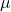and the sophisticated traders are present in measure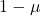. As a starting point DSSW take the safe asset S that pays a fixed dividend r. The safe asset S is in a perfectly elastic supply, which means that a unit of it can be created out of a unit of consumption good in any period and a unit can be turned back into a unit of the consumption good in any period. The price of this theoretical safe asset is fixed at 1.

The other asset, U, is an unsafe asset and pays the same fixed real dividend r as asset S. The asset U is not in a perfectly elastic supply, which means that it’s in a fixed and unchangeable quantity, normalised at one unit. The price of U, in period t, is denoted as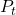.

If the price of each asset were equal to the net present value of its future dividend, then asset U and S would be perfect substituted and their prices would be the same in all periods. However, this is not how the price of U is determined in the presence of noise traders.

The sophisticated investor, in period t, accurately perceives the distribution of returns from holding the risky asset and therefore maximises the expected utility given the distribution. The noise trader, in period t, misperceives the expected price of the risky asset by an independent and identically distributed normal random variable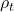.

(1)The mean perception,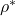, is a measure of the average ‘bullishness’ of the noise trader and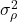is the variance of the noise traders’ misperception of the expected return per unit of the risky asset. Noise traders thus maximise their own expectation of utility given the next-period dividend, the one-period variance of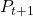and their false believe that the distribution of the price of U next period has meanabove its true value.

Each investors’ utility is a constant absolute risk aversion function of wealth when old, given by

(2)In this equation,is the coefficient of absolute risk aversion and W is the expected final wealth. Maximising U is equivalent to maximising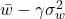, where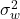is the one period ahead variance of wealth.

• The sophisticated investor chooses the amount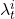of the risky asset U held to maximise

(3)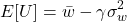(4)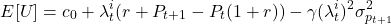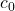is a function of first-period labor income.
• The noise trader maximises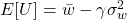as

(5)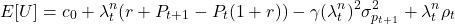The last term,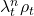, captures the noise traders’ misperception of the expected return from holding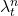units of the risky asset.

The young investors divide their portfolios between assets U and S. The quantitiesandof the risky assets are functions of its pricefor the sophisticated trader and additionalfor the noise traders. The optimal demand is a result from

(6)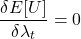• Optimal demand for risky assets from the sophisticated investor is given by

(7)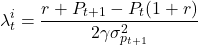• Optimal demand for risky assets from the noise investor is given by

(8)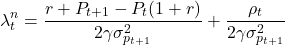The theory continues by equating the demand from the young generation to the supply from the old generation.

(9)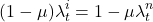(10)(11)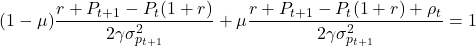This leads to the following equation regarding the price.

(12)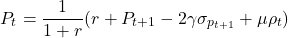(13)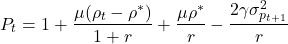The only uncertainty in the price is the noise traders’ misperception, where

(14)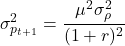Substituting Equation 14 into Equation 13 leads to

(15)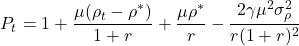Let’s discuss the terms in Equation 15.

• The second term,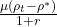, captures the fluctuation in the price of the asset U due to the variation of the noise traders’s misperceptions. When a generation of noise traders is more bullish than the average generation, they bid up the price.
• The third therm,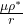, is called the price pressure effect. It captures the deviation offrom its fundamental value due to the fact that the average misperception by noise traders is not zero.
• The fourth term,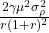, is called the create space effect. It’s a compensation for the risk, where the investors believe that the asset is mis-priced.

If noise traders’ portfolios are concentrated in assets that are subject to noise trader risk, noise traders can earn a higher average rate of return on their portfolios than sophisticated investors. Call the difference in returns between the two types of investors,

(16)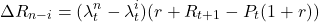The first term,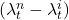, indicates the difference between the noise traders’ and sophisticated investors’ demand. This can also be written as

(17)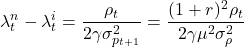The second term,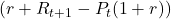, indicates the expected value of the excess return on the risky asset U. This may also be written as

(18)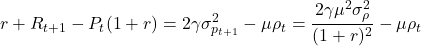(19)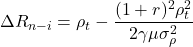Finally, as a result the given distribution in Equation 1,

(20)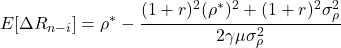Let’s take a look at the terms in Equation 20.

• The first term,, increases the noise traders’ expected returns through what might be called the hold more effect.
•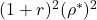incorporates the price pressure effect. As noise traders become bullish, they demand more of the risky asset on average and drive up the price. As a result, they reduce the return to risk bearing which reduces the difference in returns between the sophisticated investors and noise traders.
•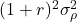incorporates the Friedmann effect, which is the risk for ‘buying high and selling low’. Because the noise traders; misperception is stochastic, they have the worst possible timing in the market. The later you step in, the more damage your poor market timing does with respect to the return.
•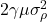incorporates the create space effect. As the variability of noise traders’ believe increases, the price risk increases. To take advantage of noise traders’ misperception, stochastic traders must bear this greater risk. However, they are risk averse, so they reduce the extend to which they bet against noise traders. If the create space effect is large, then the price pressure and Friedmann effect inflict less damage on noise traders’ average returns relative to sophisticated investors’ returns.

From this lesson, we can draw the following conclusions.

• The hold more- and create space effect tend to have a positive effect on. The Friedmann- and price pressure effect have a negative effect. Neither of the pairs dominates the equation.
• Depending on the average misperception, noise traders can have higher average returns than sophisticated traders.
• Utility of sophisticated traders is by definition higher than the utility of noise traders. The demand for unsafe assets are derived from maximising the utility function. So, the misperception creates a bias.
• The extra return does not compensate for the extra risk for noise traders, as it results in a lower utility.